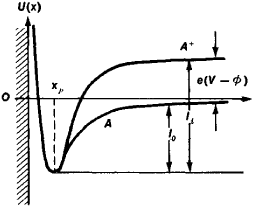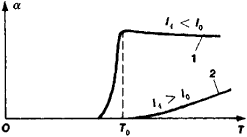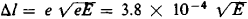# Surface Ionization

The following article is from The Great Soviet Encyclopedia (1979). It might be outdated or ideologically biased.

## Surface Ionization

the thermal desorption, or evaporation, of positive or negative ions from the surfaces of solids. To achieve a steady emission of ions in surface ionization, the rate of the corresponding ions, atoms, molecules, or radicals striking the surface (owing to the diffusion of these particles from the bulk of the solid or to adsorption occurring simultaneously with surface ionization) should be equal to the total rate of desorption of the ions and neutral particles. Surface ionization also occurs in the evaporation of the solids themselves, as in the case of refractory metals.

The measure of surface ionization is the degree of surface ionization α = vi/v0, where vi and v0 are the fluxes of the simultaneously desorbed ions and neutral particles that are identical in chemical composition; vi = CN exp (–li/kT), while v0 = DN exp (–l0/kT), where k is the Boltzmann constant, T the absolute surface temperature, li, and l0 the heats of desorption in the ionized and neutral states, and N the concentration of given particles on the surface; the coefficients C and D are weakly dependent on T in comparison with the exponents. Hence, α = (C/D) exp[(l0 - li)/kT].Figure 1. Potential curves for the interaction of two systems: (1) the surface of a solid body and the neutral particle (A); (2) the surface and the positive ion (A+). The quantity x is the distance from the surface, and U(x) is the energy bonding the particle with the surface. The distance x1,, corresponds to the equilibrium state of the particle at the surface, and the depths of the potential wells ll and l0 are equal to the heats of desorption of the ion and neutral particle, respectively. The difference ll–l0 is equal here to the difference between the ionization energy eV of the neutral particle (V is the particle’s ionization potential, and e the electron charge) and the surface work function .

The interaction of the particles with the surfaces is represented by curves of the type shown in Figure 1. The transition from the curve for the neutral particles A to the curve for the ions A+ at a distance x → ∞ from the surface corresponds to the ionization of the particle with the transfer of the freed electron to the solid. The energy required for this process is equal to e(V —Φ), where V is the ionization potential of the particle, the work function of the solid, and e the charge of an electron. Expressing α in terms of these quantities the Langmuir-Shaha equation is obtained. For positive surface ionization, (li+ — l0) = e(V — Φ), and for negative surface ionization, (li_ — l0) = e (Φ —S), where eS is the electron affinity energy of the particle.

Surface ionization is most effective (ɑ is large) for particles with li, < l0 or Φ > V and S > Φ; for these particles, α decreases as Tincreases. For inverse inequalities, surface ionization becomes intensified as T increases (Figure 2). The quantities li and l0 depend on N; li, usually increases and l0 decreases as N increases. When T > T0, the condition of efficient surface ionization— li < lo and vi > v0— holds, whereas when T = To, the sign of the term (l0 — li) changes and α begins sharply decreasing to low values. T0 is called the temperature threshold of surface ionization.Figure 2. Characteristic dependence of the degree of surface ionization in steady surface ionization on temperature T: (1) when the heat of desorption of the ion li is less than the heat of desorption of the neutral particle l0; (2) when li >l0. T0 is the temperature threshold of surface ionization.

An external electric field E that accelerates the ions from the surface reduces the value of li. When E< 107 V/cm, this reduction ∆l eelectron volts (E should be expressed in V/cm); α increases correspondingly. When li < l0 and vi > v0, E at steady surface ionization reduces N and T0 Thus, T0 for cesium atoms on tungsten when E =104 V/cm is 1000°K and is reduced to 200°K when E = 107 V/cm. This allows the phenomena of desorption and evaporation of ions by an electric field at low temperatures to be considered as surface ionization. Modern experimental technology permits the observation of surface ionization of particles with V ≤ 10 V and S ≥ 0.6 V. With an electric field, these limits may be considerably expanded.

These principles of surface ionization have been confirmed by experiments for uniform surfaces. However, in practice, nonuniform surfaces are most often encountered. On nonuniform surfaces, l0, li, Φ and N are different in different spots. In such cases, the indicated dependence of α on T and E hold only for some averaged values of lo, li, and Φ

Surface ionization is widely used in ion sources of various kinds, in sensitive particle detectors, and when compensating for the space charge of electrons in thermionic converters. It is a promising method for producing plasma engines, as well as a foundation for investigating the physicochemical characteristics of the surfaces of solids and of the particles interacting with these solids.

### REFERENCE

Zandberg, E. Ia., and N. I. Ionov. Poverkhnostnaia ionizatsiia. Moscow, 1969.

N. I. IONOV